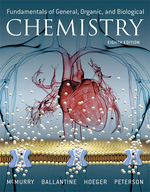×

×

# Solutions for Chapter 21: The Generation of Biochemical Energy## Full solutions for Fundamentals of General, Organic, and Biological Chemistry (Mastering Chemistry) | 8th Edition

ISBN: 9780134015187Solutions for Chapter 21: The Generation of Biochemical Energy

Solutions for Chapter 21
4 5 0 283 Reviews
31
0
##### ISBN: 9780134015187

Summary of Chapter 21: The Generation of Biochemical Energy

This expansive textbook survival guide covers the following chapters and their solutions. Fundamentals of General, Organic, and Biological Chemistry (Mastering Chemistry) was written by Aimee Notetaker and is associated to the ISBN: 9780134015187. Since 13 problems in chapter 21: The Generation of Biochemical Energy have been answered, more than 1643 students have viewed full step-by-step solutions from this chapter. Chapter 21: The Generation of Biochemical Energy includes 13 full step-by-step solutions. This textbook survival guide was created for the textbook: Fundamentals of General, Organic, and Biological Chemistry (Mastering Chemistry), edition: 8.

Key Chemistry Terms and definitions covered in this textbook
• Aceto group

A CH3CO! group; also called an acetyl group

Reactions that are characterized by the addition of two groups across a double bond. In the process, the pi (p) bond is broken.

• alloy

A substance that has the characteristic properties of a metal and contains more than one element. Often there is one principal metallic component, with other elements present in smaller amounts. Alloys may be homogeneous or heterogeneous. (Section 12.3)

• Arrhenius equation

An equation that relates the rate constant for a reaction to the frequency factor, A, the activation energy, Ea, and the temperature, T: k = Ae-Ea>RT. In its logarithmic form it is written ln k = -Ea>RT + ln A. (Section 14.5)

• Atropisomers

Enantiomers that lack a chiral center and differ because of hindered rotation.

• barometer

An instrument that measures atmospheric pressure. (5.2)

• collision model

A model of reaction rates based on the idea that molecules must collide to react; it explains the factors influencing reaction rates in terms of the frequency of collisions, the number of collisions with energies exceeding the activation energy, and the probability that the collisions occur with suitable orientations. (Section 14.5)

• coordinate covalent bond.

A bond in which the pair of electrons is supplied by one of the two bonded atoms; also called a dative bond. (9.9)

• cycloalkanes

Saturated hydrocarbons of general formula CnH2n in which the carbon atoms form a closed ring. (Section 24.2)

• electrocyclic reaction

A pericyclic process in which a conjugated polyene undergoes cyclization. In the process, one p bond is converted into a s bond, while the remaining p bonds all change their location. The newly formed s bond joins the ends of the original p system,thereby creating a ring.

• Equatorial bond

A bond to a chair conformation of cyclohexane that extends from the ring roughly perpendicular to the imaginary axis through the center of the ring; a bond that lies roughly along the equator of a cyclohexane ring

• Glycoside

A carbohydrate in which the !OH on its anomeric carbon is replaced by !OR

• greenhouse gases

Gases in an atmosphere that absorb and emit infrared radiation (radiant heat), “trapping” heat in the atmosphere. (Section 18.2)

• heat of fusion

The enthalpy change, ?H, for melting a solid. (Section 11.4)

• heterocycle

A cyclic compound containing at least one heteroatom (such as S, N, or O) in the ring.

• lattice points

Points in a crystal all of which have identical environments. (Section 12.2)

• molecular-orbital theory

A theory that accounts for the allowed states for electrons in molecules.(Section 9.7)

• oxirane

A cyclic ether containing a three-membered ring system. Also called an epoxide.

• sulfonation

An electrophilic aromatic substitution reaction in which an SO3H group is installed on an aromatic ring.

• symmetry forbidden

A reaction that disobeys conservation of orbital symmetry.